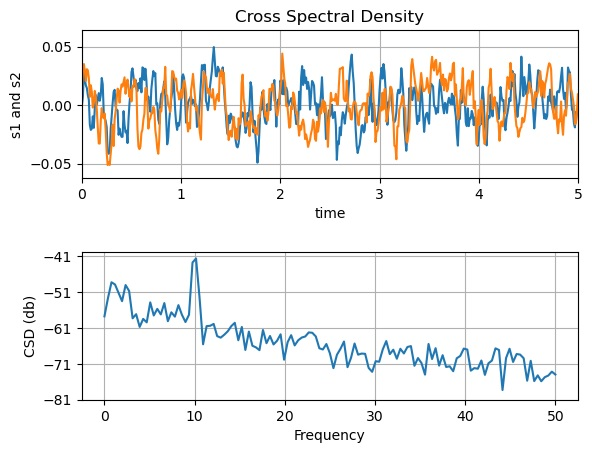# Cross Spectral Density in Python using Matplotlib

In this tutorial, we are going to plot cross spectral density in python using matplotlib.
Submitted by Anuj Singh, on July 30, 2020

CSD (cross-spectral density) is often used in signal processing and therefore, we are presenting an example for the same.## Python code for cross spectral density using matplotlib

```import numpy as np
import matplotlib.pyplot as plt

fig, (ax1, ax2) = plt.subplots(2, 1)

dt = 0.01
t = np.arange(0, 30, dt)

nse1 = np.random.randn(len(t))
nse2 = np.random.randn(len(t))
r = np.exp(-t / 0.05)

cnse1 = np.convolve(nse1, r, mode='same') * dt
cnse2 = np.convolve(nse2, r, mode='same') * dt

s1 = 0.01 * np.sin(2 * np.pi * 10 * t) + cnse1
s2 = 0.01 * np.sin(2 * np.pi * 10 * t) + cnse2

ax1.plot(t, s1, t, s2)
ax1.set_xlim(0, 5)
ax1.set_xlabel('time')
ax1.set_ylabel('s1 and s2')
ax1.set_title('Cross Spectral Density')
ax1.grid(True)

cxy, f = ax2.csd(s1, s2, 256, 1. / dt)
ax2.set_ylabel('CSD (db)')
plt.show()
```

Output:

```Output is as figure
```